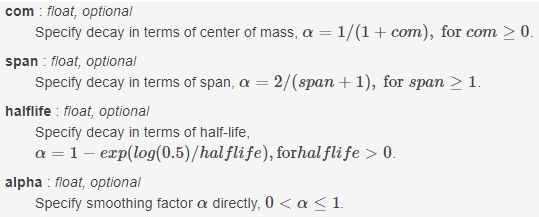# pandas 学习 第3篇：Series - 数据处理（应用、分组、滚动、扩展、指数加权移动平均）

## 一，应用和转换函数

### 应用apply

Series.apply(self, func, convert_dtype=True, args=(), **kwds)

• func：应用的函数，可以是自定义的函数，或NumPy函数
• convert_dtype：默认值是True，尝试把func应用的结果转换为更好的数据类型，如果设置为False，把结果转换为dtype=object.
• args：元组，在序列值之后，传递给func的位置参数（positional arguments）
• **kwds：传递给func的关键字（keyword）参数，可以有0、1、多个

• 位置参数是通过匹配位置来传参，关键字参数是通过匹配参数名称来传参。
• 关键字参数可以有多个，参数的名称不固定，只能在apply函数()的最后面，例如，关键字参数k1,k2,k3，那么kwargs=[k1,k2,k3]
• 位置参数args只能有一个

1，传递自定义的函数（使用位置参数）

>>> s = pd.Series([20, 21, 12], index=['London', 'New York', 'Helsinki'])
>>> def subtract_custom_value(x, custom_value):
...     return x - custom_value
>>> s.apply(subtract_custom_value, args=(5,))
London      15
New York    16
Helsinki     7
dtype: int64

2，传递自定义的函数（使用关键字参数）

>>> def add_custom_values(x, **kwargs):
...     for month in kwargs:
...         x += kwargs[month]
...     return x
London      95
New York    96
Helsinki    87
dtype: int64

### 转换transform

Series.transform(self, func, axis=0, *args, **kwargs)

## 二，分组

Series.groupby(self, by=None, axis=0, level=None, as_index=True, sort=True, group_keys=True, squeeze=False, observed=False, **kwargs)

by：用于对序列进行分组，参数by的值可以是函数，列名或列名列表，映射

1，by是函数

>>> s=pd.Series([1,2,3,4])
>>> s.groupby(by=lambda x: x<3).count()
False    1
True     3
dtype: int64

>>> s.groupby(by=lambda x: s.iat[x]<3).count()
False    2
True     2
dtype: int64

2，by是标签列表

3，映射（字典）

>>> s.groupby(by={1:'a',2:'a',3:'b',4:'b'}).count()
a    2
b    1
dtype: int64

4，映射（序列）

>>> s.groupby(by=pd.Series(data=[1,2,1,1],index=[0,2,3,1])).mean()
1    2.333333
2    3.000000
dtype: float64

by序列的索引是0, 2, 3, 1，也就是说，当原始序列的索引为0, 3, 1 时，对应的分组key是1，当原始序列的索引为2时，对应的分组key是2。

## 三，滚动

Series.rolling(self, window, min_periods=None, center=False, win_type=None, on=None, axis=0, closed=None)

• window：滚动的窗口值，或偏移量，每一个窗口都是一个固定值。
• min_periods：每个窗口的最小值，如果窗口中的元素数量小于min_periods，返回NaN；默认情况下，min_periods等于window参数的值。

>>> s=pd.Series([1,2,3,4])
>>> s.rolling(2).sum()
0    NaN
1    3.0
2    5.0
3    7.0
dtype: float64
>>> s.rolling(window=2,min_periods =1).sum()
0    1.0
1    3.0
2    5.0
3    7.0
dtype: float64

## 四，扩展

Series.expanding(self, min_periods=1, center=False, axis=0)

>>> s=pd.Series([1,2,3,4])
>>> s.expanding().sum()
0     1.0
1     3.0
2     6.0
3    10.0
dtype: float64

## 五，指数加权移动平均

ewm（Exponentially Weighted Moving）是指数加权移动的简称，通常情况下，是对序列的元素进行指数加权，计算加权后的均值：

Series.ewm(self, com=None, span=None, halflife=None, alpha=None, min_periods=0, adjust=True, ignore_na=False, axis=0)

1，参数注释• 当设置adjust为True时，加权均值的计算公式是： (1-alpha)**(n-1), (1-alpha)**(n-2), …, 1-alpha, 1
• 当设置adjust为False时，加权均值的计算公式是：weighted_average = arg; weighted_average[i] = (1-alpha)*weighted_average[i-1] + alpha*arg[i].

2，指数加权移动平均的意义

>>> s=pd.Series([1,2,3,4])
>>> s.ewm(alpha=0.8).mean()
0    1.000000
1    1.833333
2    2.774194
3    3.756410
dtype: float64
>>> s.ewm(alpha=0.2).mean()
0    1.000000
1    1.555556
2    2.147541
3    2.775068
dtype: float64

pandas.Series.apply

posted @ 2019-09-24 10:59  悦光阴  阅读(1349)  评论(0编辑  收藏